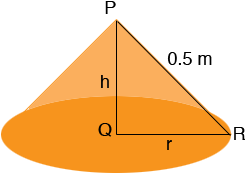SEARCH HOMEMath Central Quandaries & QueriesQuestion from lee, a student: hi i have a cone to calculate, the height and radius are equal and the slant height is 0.5m the total surface area is 15m sq i need to calculate the radius of the cone Note : this will lead to a quadratic equation could somebody have a look please Thanks LeeHi Lee,

There is something incorrect in this problem. If the height h and radius r are equal then the situation you have isSince triangle PQR is a right triangle, Pythagoras' theorem tells us that

h2 + r2 = 0.52

But r = h so

2 r2 = 0.52 or r2 = 0.125 and r = 0.35 meters

PennyMath Central is supported by the University of Regina and The Pacific Institute for the Mathematical Sciences.